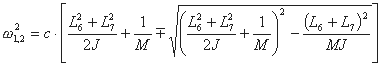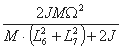# MasterAllRound: the PC calculator

## Example: eigenfrequency computation

This example shows how to determine the Eigenfrequencies of a sewing machine. We assume that you are familiar with the topic, but want to know how to solve the mathematical side with MasterAllRound. Here is a short version of an example from the manual showing some additional options in MasterAllRound:

You obtain the Eigenfrequencies by solving the following equation:with c defined as:Input data are:
• L6 = 220 mm
• L7 = 120 mm
• M = 18 kg (mass of the machine)
• J = 0.18 kgm2 (mass moment of inertia)
• n = 1000 min-1 (nominal speed)
Units are [N], [mm] and [s]. First, we enter the above constants. Note that you can add comments when defining constants in MasterAllRound:
• L6 = 220      ' [ mm]
• L7 = 120      ' [ mm]
• M = 18/1000      ' [N*s^2/mm]
• J = 0.18E3      ' [N*mm*s^2]
• OMI = 2*PI*1000/60      ' n = 1000 min^(-1)
We rewrite the equation using sub-equations:
• TC = 2*J*M*OMI^2/(M*(l6^2+L7^2)+2J)
• TG1 = (L6^2+L7^2)/(2J) + 1/M
• TG2 = (L6+L7)^2/(M*J)
That's all on the set-up side: we can solve the task by entering in the formula input field our equation:
• f1 = (SQR(TC*(TG1-SQR(TG1^2-TG2))))/(2*PI)      ' @
The "@" indicates that we are using sub-equations. Finalizing our input with the return key, or clicking on the "Run!" button, gives us the result:
• f1 = 10.9219 [Hz]
To obtain the second solution, we just edit the equation in the input field:
• "f1" in "f2"
• "-SQR" in "+SQR"
The second equation is:
• f2 = (SQR(TC*(TG1+SQR(TG1^2-TG2))))/(2*PI)      ' @
We finalize our input with the return key and obtain the second frequency:
• f2 = 20.887 [Hz]It's very easy to investigate changes in the Eigenfrequencies now. For example: all we have to do, to study how f1 changes as a function of M, is to define M as a variable. As a result, we obtain ordered pairs of M and f1. We can also plot this by saving the table and using the plot module of MasterAllRound.